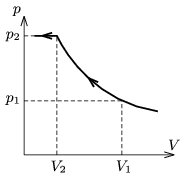Mathematical and Physical Journal
for High Schools
Issued by the MATFUND Foundation
 Already signed up? New to KöMaL?

#Problem P. 4334. (March 2011)

P. 4334. In a container which is equipped with a piston there is a mixture of Oxygen and Nitrogen gas at a temperature of T=77.4 K. Keeping the temperature constant the mixture is slowly compressed. The pressure of the mixture as a function of the volume changes as shown in the figure, where V1=15 dm3 és p1=56.3 kPa.

a) How much Oxygen and Nitrogen are there in the container?

b) Determine thecoordinates of that single point on the isotherm, where the graph has a cusp.(5 pont)

Deadline expired on April 11, 2011.

Sorry, the solution is available only in Hungarian. Google translation

Megoldás. $\displaystyle a)$ 1,07 mol nitrogén és 0,24 mol oxigén van a tartályban.

$\displaystyle b)$ $\displaystyle p_2=$123,8 kPa; $\displaystyle V_2=$6,82 $\displaystyle \rm{dm}^3$.

### Statistics:

 37 students sent a solution. 5 points: Agócs Fruzsina, Béres Bertold, Bolgár Dániel, Czigány Máté Gábor, Czipó Bence, Filep Gábor, Fülep Andrea , Janzer Olivér, Jenei Márk, Juhász Péter, Koncz Gabriella, Kovács 444 Áron, Laczkó Zoltán Balázs, Maknics András, Nagy 111 Miklós, Pataki Bálint Ármin, Pázmán Koppány, Pető János, Sárvári Mátyás, Simon Sebestyén, Szabó 928 Attila, Szikszai Lőrinc, Várnai Péter, Vuchetich Bálint, Zsámboki Richárd. 2 points: 1 student. 1 point: 9 students. 0 point: 2 students.

Problems in Physics of KöMaL, March 2011